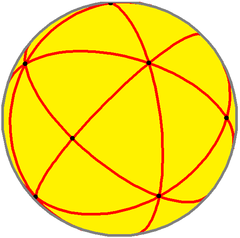We will now discuss the octahedron and its associated Archimedean and Catalan solids.

The Octahedron – 1440º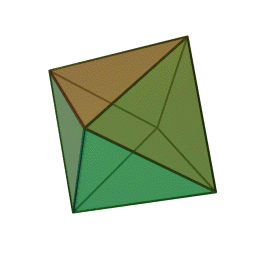It has:

• 8 Faces; four meeting at every vertex
• 12 Edges
• 6 Corners

It is composed of two pyramids of square base.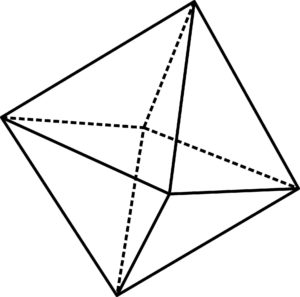The diagonal through the octahedron (the diagonal of the square base) will equal √2 if the side lengths are 1.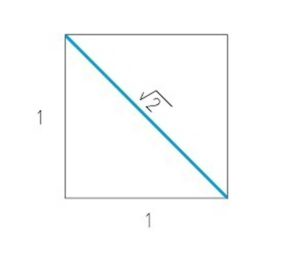The octahedron is related to “Air” by Plato.  It is considered it an intermediary between Fire (tetrahedron) and Water (icosahedron).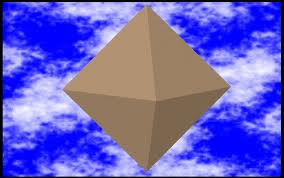The Dual of the Octahedron is the Cube.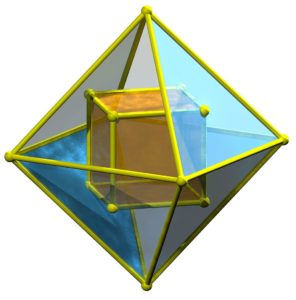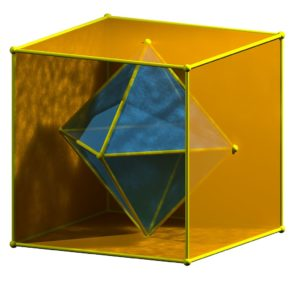The Octahedron is also a rectified tetrahedron.  Remember, fully truncating (rectifying) the tetrahedron produces the octahedron.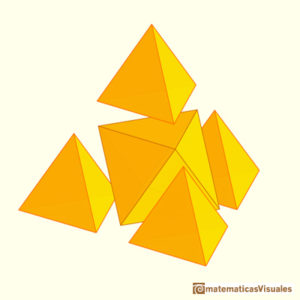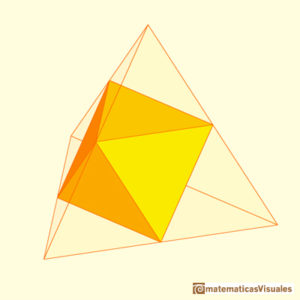Projections of the Octahedron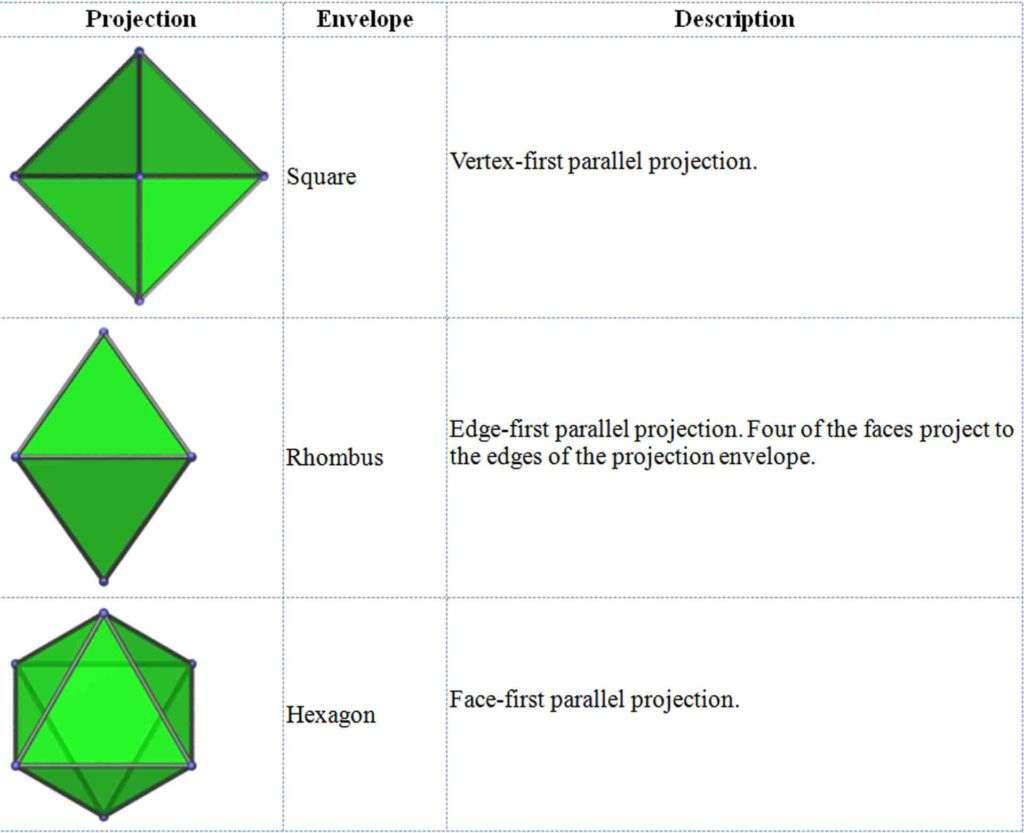The Octahedron Has:

• Six 2-fold axes passing through opposite edges
• Four 3-fold axes passing through its face centers
• Three 4-fold axes passing through opposite vertices

Six Close-packed Spheres Make an Octahedron: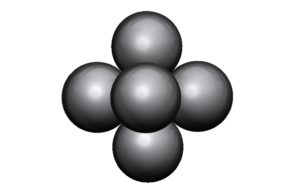The cirumradius is larger than its inradius by a factor of √3.

Here is an octahedron with its insphere: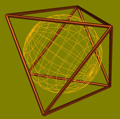Here is an octahedron with its circumsphere: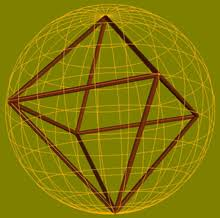The Octahedron in Metatron’s Cube: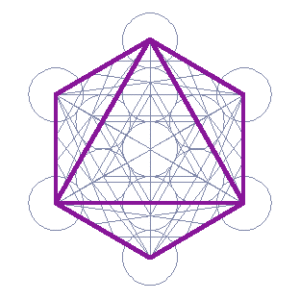1440°

The Sum of its Angles = 1440°.  This is the same as the Star Tetrahedron.

Recall the Importance of the Number 1440:

1440 hertz = F# (This is one octave up from a tetrahedron)

• 1440 Minutes in a day
• 144 inches/foot
• There are 14400 total degrees in the five Platonic solids.

• 122 = 12 x 12 = 144
• 12 Disciples of Jesus & Buddha
• 12 circles clustering around 1 (Fruit of Life)
• 12 spheres clustering around 1 (Cuboctahedron)
• 12 Constellations or Houses of the Zodiac
• 12 Ages of the Precession of the Equinoxes
• 12 Hours on a clock face

• 144,000 will be saved in the Rapture prior to the Apocalypse according to the Christian Bible. Please note this is not literal.  It is symbolic!
• The Wall surrounding the Holy City in Revelations = 144 cubits.
• 144,000 smooth white casing stones once covered the Great Pyramid.
• 144,000 days = 1 Mayan baktun (394.26 years).
• 144 cubits = 216 feet (x 10) = 2160 = the diameter of the moon in miles.

• Earth turns on its axis 24 hours:
• 24 hours x 60 minutes = 1440 minutes/day
• 1440 x 60 seconds = 86400 seconds/day
• 86400 (x 10) = 864000 = diameter of the Sun

Spherical Octahedron: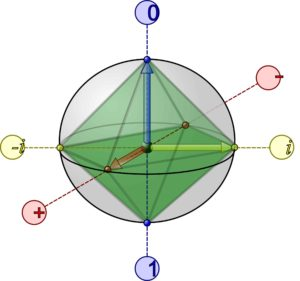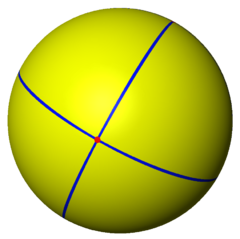Net of the Octahedron: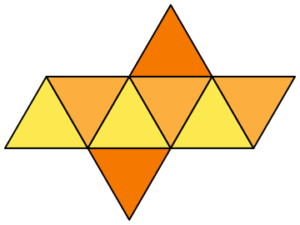Like the cube, there are 11 different nets, or configurations of 8 equilateral triangles, that produce the octahedron.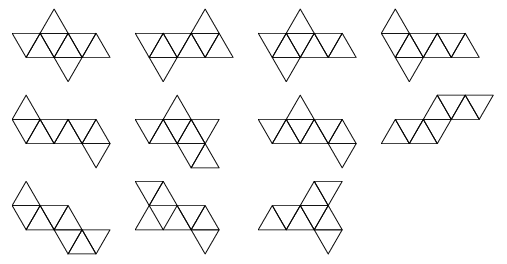Volume and Surface Area of the Octahedron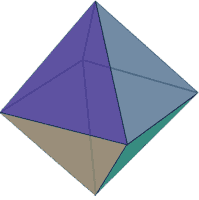Volume = √2/3 s3                                s = side length

Surface Area = 2√3s2                            s = side length

As Buckminster Fuller teaches, the octahedron falls in the middle of the tetrahedron and icosahedron in terms of volume efficiency and load resistance.  “It will ‘dimple’, but in doing so one half caves in to ‘nest’ exactly inside the other half.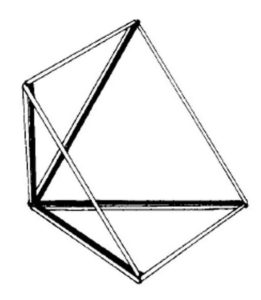The tetrahedron will not dimple.  It is very stable.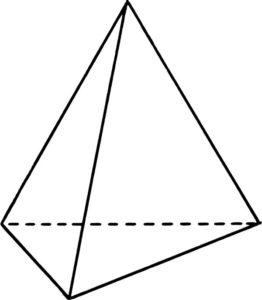The icosahedron dimples very easily.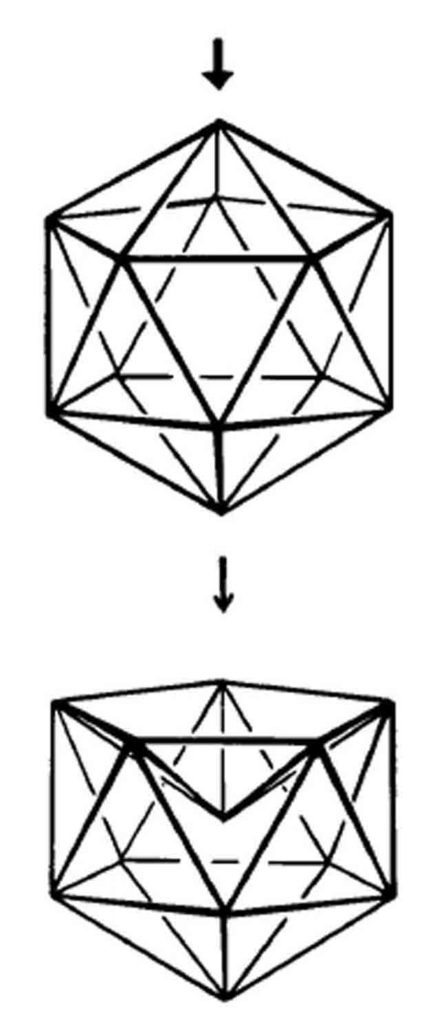The octahedron falls in the middle of the two.

As discussed many times before, the stellated octahedron, or star tetrahedron is found as the structure of the photon.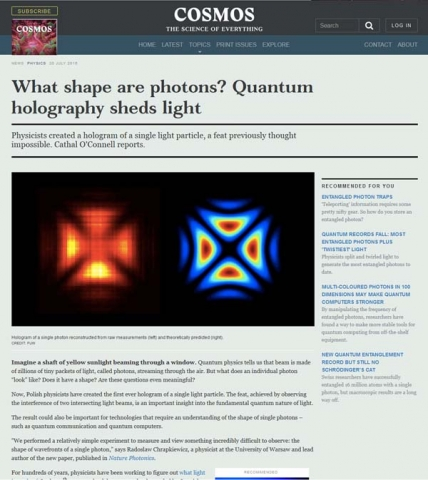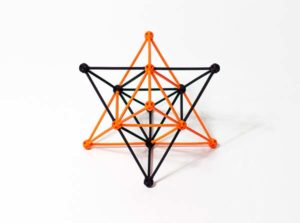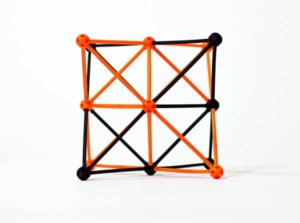The structure of the photon is a stellated octahedron (star tetrahedron) seen from its ‘cubic’ angle.

In Dr. Robert Moon’s work, the octahedron is the structure of the element silicon.  There are 8 points of the cube + 6 points of the octahedron = 14.  14 points = 14 protons.  The cube is within the octahedron.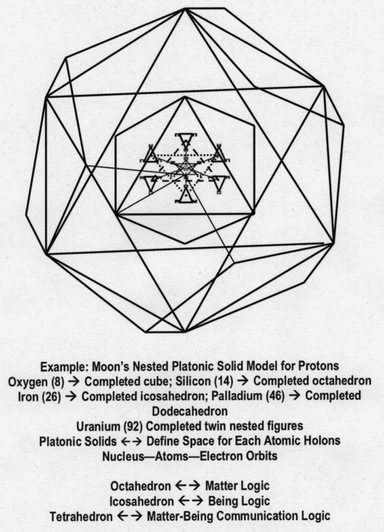The Octahedron is also commonly found in the structure of molecules.

Six pairs of electrons in the valence shell (outer shell) form an octahedron.

Angles of molecular bonding patterns include centered octahedral at 90º and not centered octahedral at 60º and 90º.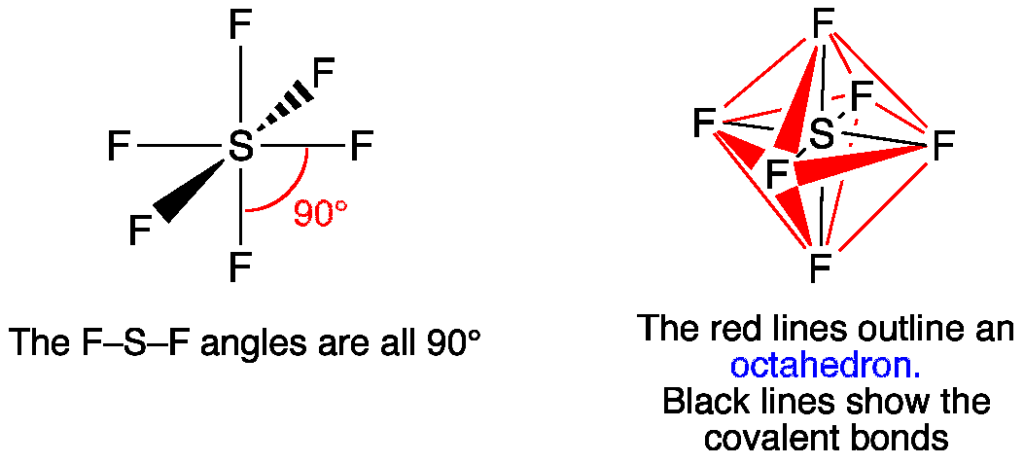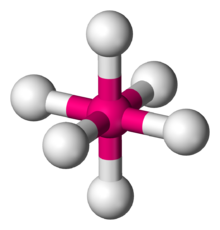Clay is composed of an octahedral sheet sandwiched between two tetrahedral sheets. These are the layers of tetrahedrally arranged silicate and octahedrally arranged aluminate groups.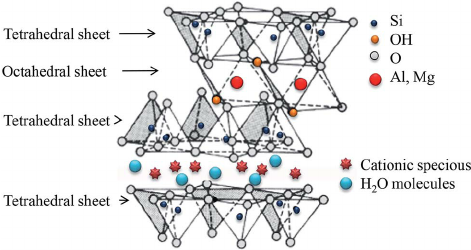The Octahedron is also found in the mineral kingdom: mineral diamonds, fluorite & spinel crystals often form octahedra.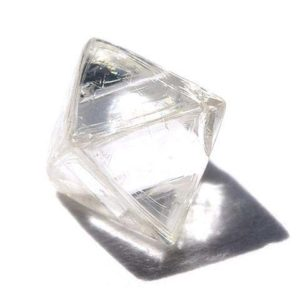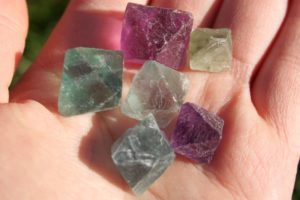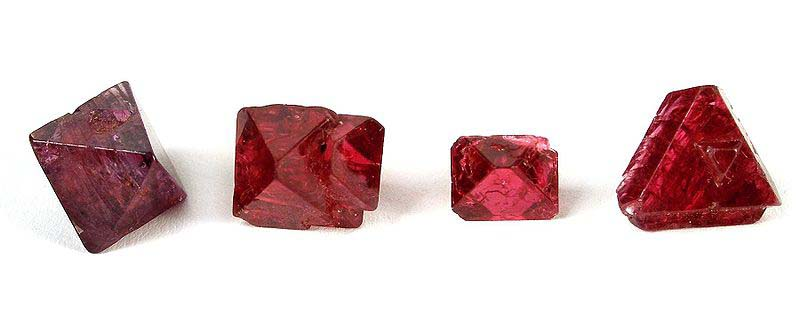Several radiolaria (zooplankton) are structured on the octahedron.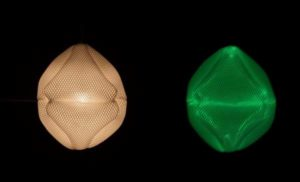Tentaculus Giganticus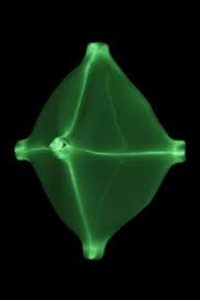Hexapodus Inflatus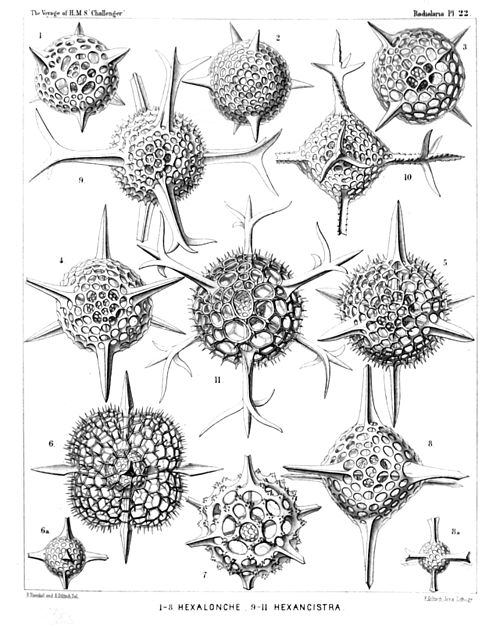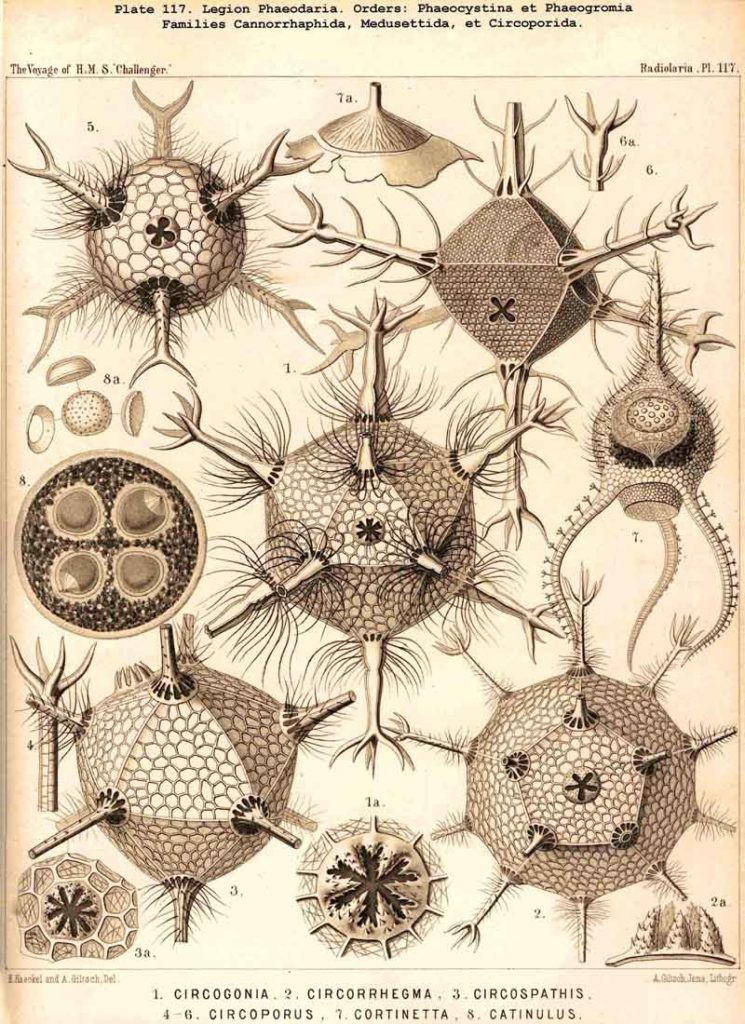The Octahedron is also found in the large-scale structure of the universe.

In the work of Battaner and Florido in 1997 they found evidence of an octahedral structure in galactic clustering.  They call this the “egg carton” universe.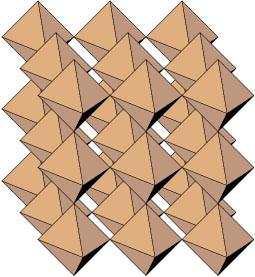In this model there would be larger amounts of matter along the edges of the octahedra, which would be sites of the superclusters.  Outside the filaments there would be large voids.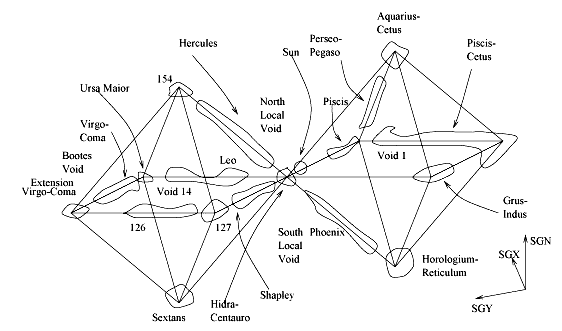In the work of Conrad Ranzan and the Dynamic Steady State Universe (DSSU) the Cosmic Gravitation Cells are composed of tetrahedra and octahedra.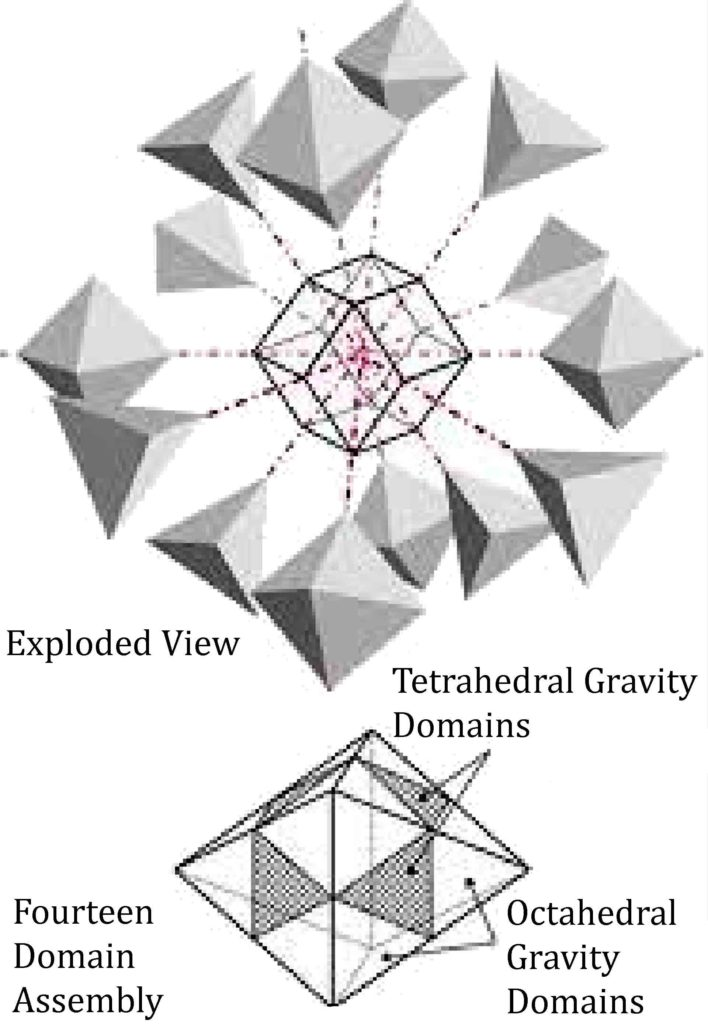The minor nodes are tetrahedra and the major nodes are octahedra.  These fit together to form a super-octahedron.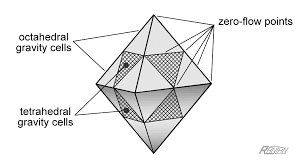All these scientific concepts regarding the Platonic solids are discussed in great detail in the Science section of in Cosmic Core.

The Octahedron & Tetrahedron

An octahedron nests within a tetrahedron.The 6 edge midpoints of the tetrahedron define the 6 vertices of the octahedron.

The octahedron halves the tetrahedron’s edges.

The octahedron halves the tetrahedron’s surface area & volume.

This embodies the octave 1:2.

Rectification of the Tetrahedron and the Octahedron

Remember, truncating the tetrahedron in its extreme creates the octahedron.  This is called rectification.

In other words, the rectification of the tetrahedron creates the octahedron.The Tetrahedral-Octahedral Honeycomb or Tessellation

Alternating octahedra and tetrahedra can tessellate, or fill space, in a ratio of 1:2, that is 2 tetrahedra to every 1 octahedra.  This is the same structure as the cosmic gravitation cells of Conrad Ranzan and the Dynamic Steady State Universe Model.Below is an image of what the “Super-Octahedron” would look like.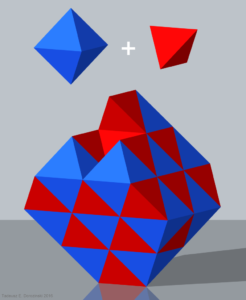The Stellated Octahedron:  The Star Tetrahedron & Octahedron

There is only one stellation of the octahedron.  This produces the star tetrahedron.

That means, putting a tetrahedron onto each face of the octahedron produces a star tetrahedron (stella octangula), two interlocking tetrahedra.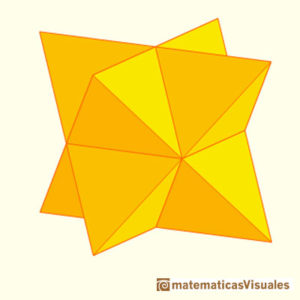The volume enclosed by the two interlocking tetrahedra defines an octahedron.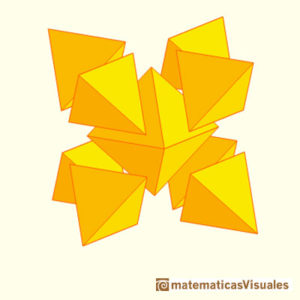That means an octahedron is always present inside a star tetrahedron.  When the star tetrahedron is pointed upwards (as seen above on the right) then the octahedron is tilted at a 45º angle.

The Octahedron and the Cube

The Octahedron and Cube are Duals.  Connecting the centers of faces creates the dual solid.The octahedron’s points are truncated to form the cube.

The intermediary stage between the transition between the octahedron and cube produces the cuboctahedron, an Archimedean solid we will cover in great detail in the next article.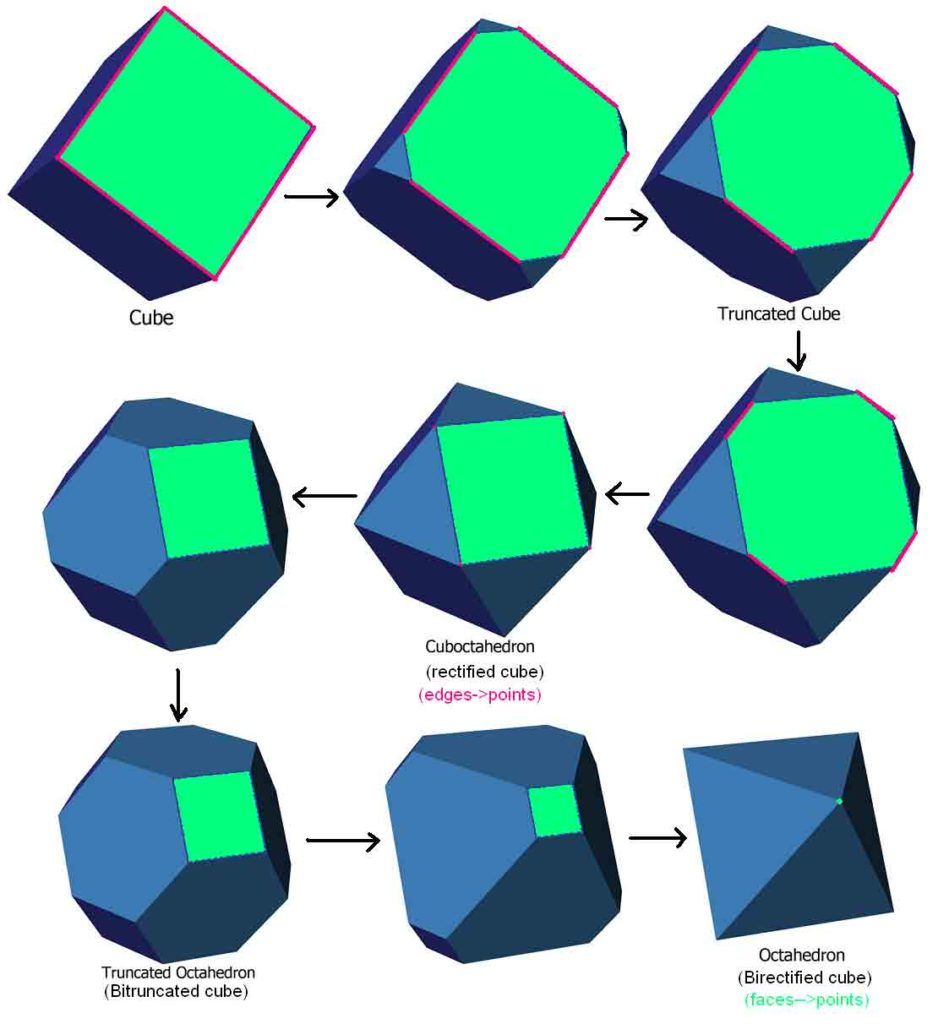The Octahedron and the Icosahedron

Each of the 12 edges of the octahedron correspond to the 12 vertices of the icosahedron.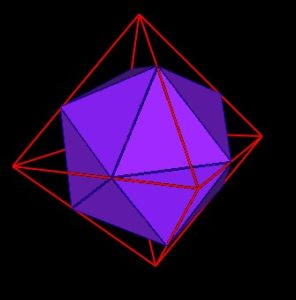The icosahedron’s vertices cut the octahedron’s edges perfectly in the Golden Section.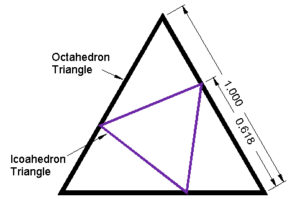It is interesting to note here that the octahedron and icosahedron are different phases of the Jitterbug motion.  Additionally, the 8 triangles of the Jitterbug pass through a regular dodecahedron phase.

“If the position of one of the Jitterbug triangles in the dodecahedron position in drawn in the triangular face of an octahedron, we get the following diagram:”4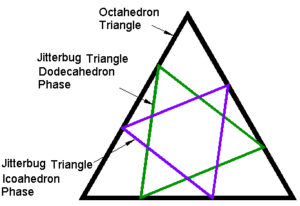Icosahedron to Octahedron

By angularly tilting the icosahedron on its side (we have not calculated the exact number of degrees of tilt that are necessary,) and adding a special harmonic tetrahedral shape in twelve different places, we can build the octahedron.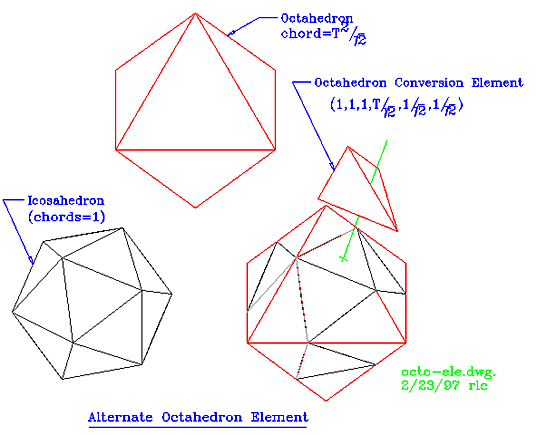Credit: David Wilcock

We saw above that the stellated octahedron is the same as the star tetrahedron.  Now we will look at the truncated octahedron.

Truncated Octahedron & Dual Tetrakis Hexahedron

We will now discuss an Archimedean solid, the Truncated Octahedron and its dual, the Tetrakis Hexahedron, a Catalan solid.

Truncated Octahedron – 7920º

The truncated Octahedron is formed by removing six right square pyramids from the corners of the octahedron – one from each point.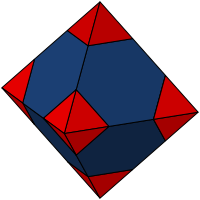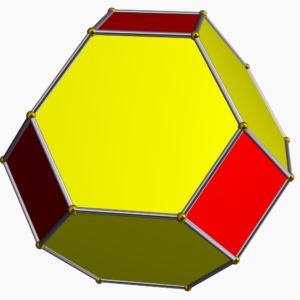It has:

• 14 Faces (8 regular hexagons; 6 squares)
• 36 Edges
• 24 Vertexes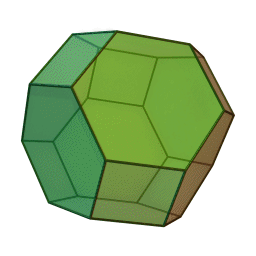The Sum of its Angles = 7920º

Projections of the Truncated Octahedron

Here are some commonly viewed angles: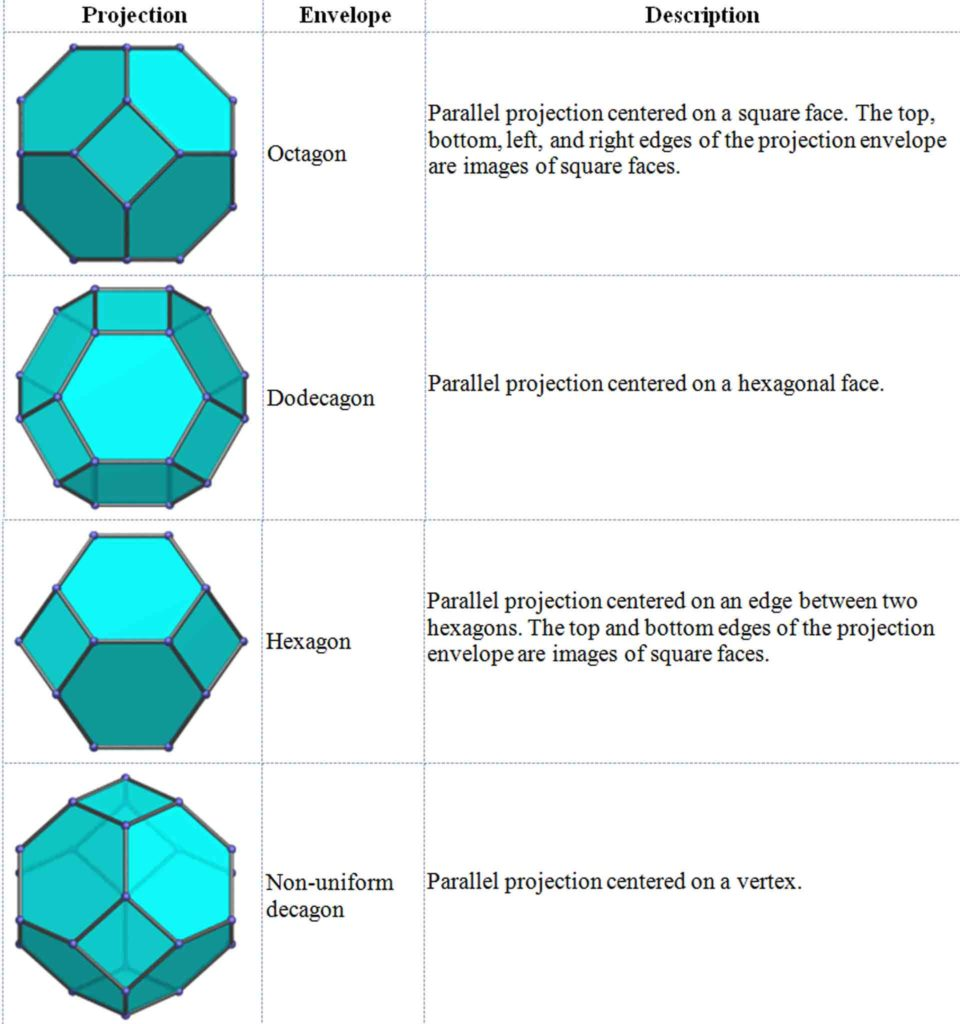The Spherical Truncated Octahedron: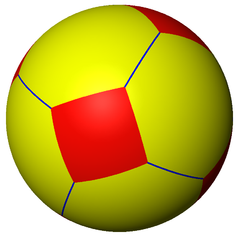Stereographic Projections of the Truncated Octahedron: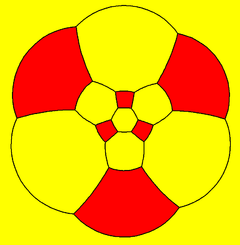Net of the Truncated Octahedron: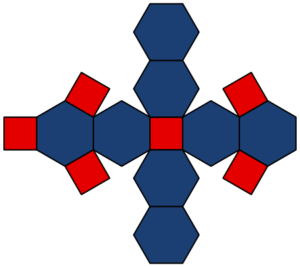Area and Volume of the Truncated Octahedron

Area = (6 + 12√3) s2                           s = side length

Volume = 8√2s3                                  s = side length

A truncated tetrahedron can also be built by 8 half cubes.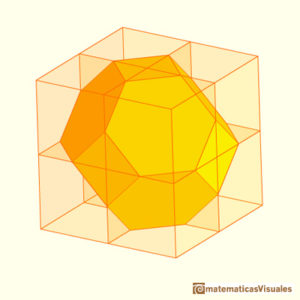The Truncated Octahedron can tessellate in space, like the cube.  There will be no gaps.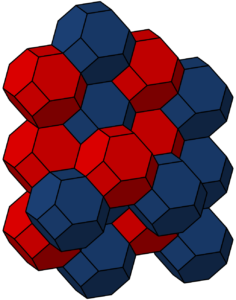The Truncated Octahedron exists in the structure of faujasite crystals.  These crystals are in a mineral group in the zeolite family of silicate minerals.  It occurs naturally worldwide, though it is rare.  It is also synthesized industrially to be used in the petroleum industry.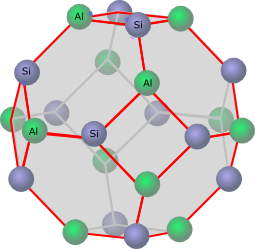Tetrakis Hexahedron – 4320º

The Tetrakis Hexahedron is a Catalan solid, the dual of the truncated octahedron.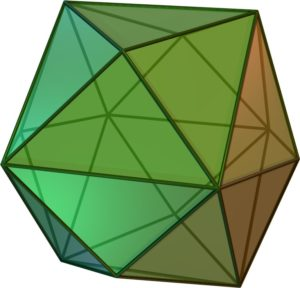It has:

• 24 Faces (isosceles triangles)
• 36 Edges
• 14 Vertices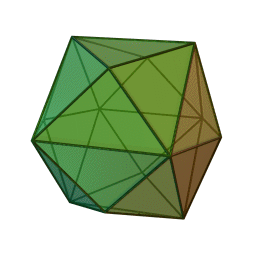The faces look as follows: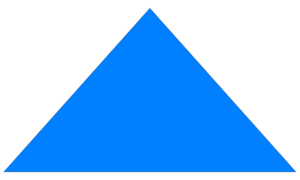The Sum of its Angles = 4320º.

It can also be seen as a cube with square pyramids covering each square face.  Hence it is a Kleetope of the cube.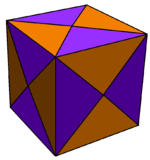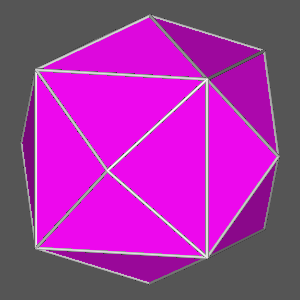Net of the Tetrakis Hexahedron: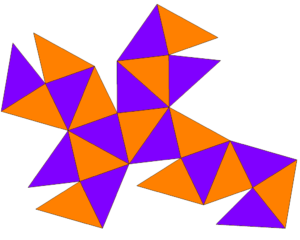The Projections of the Tetrakis Hexahedron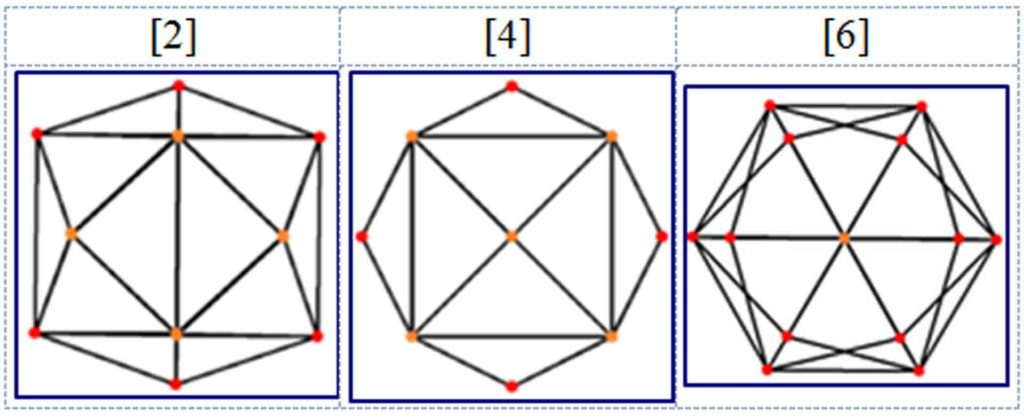“Naturally occurring crystal formations of tetrahexahedra are observed in copper and fluorite systems.”1

The Spherical Tetrakis Hexahedron: